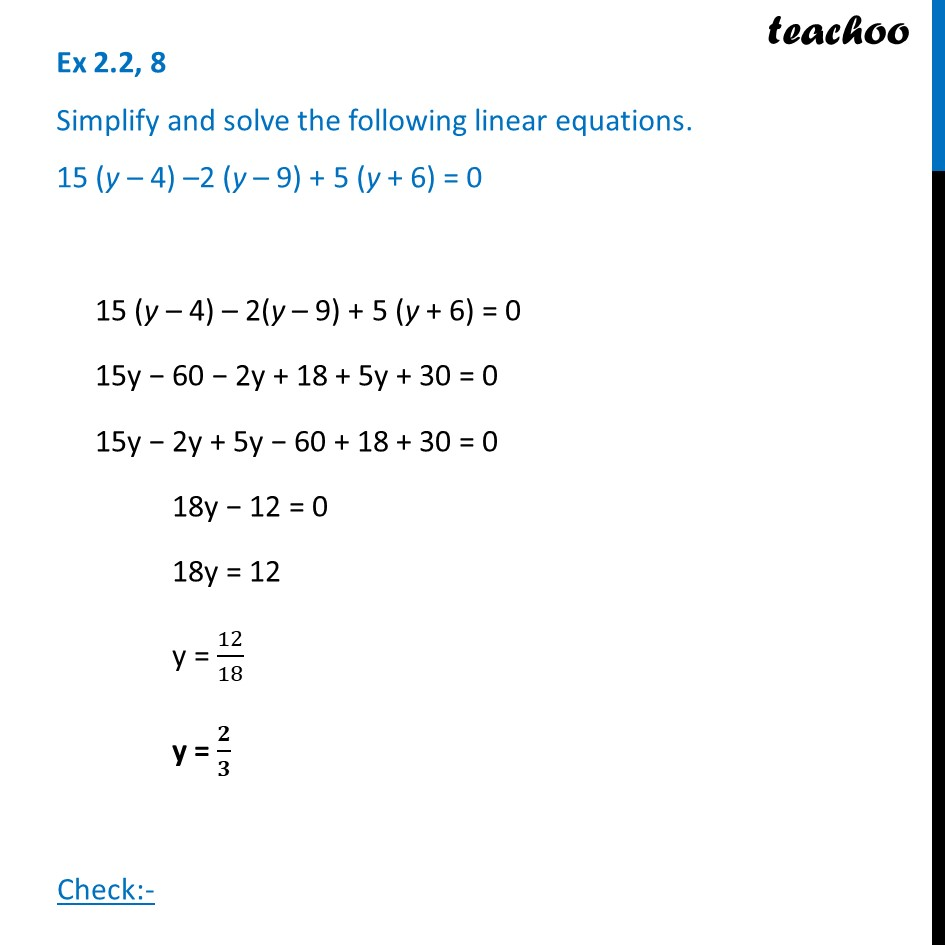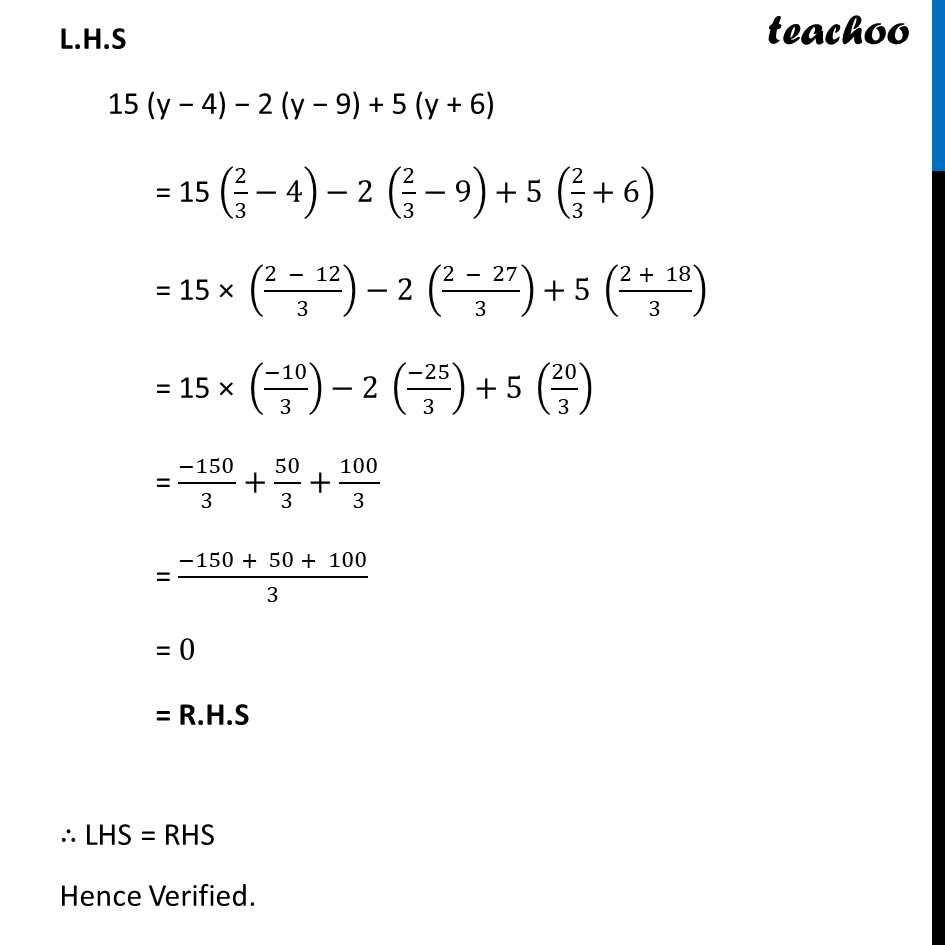Ex 2.2

Chapter 2 Class 8 Linear Equations in One Variable
Serial order wiseLearn in your speed, with individual attention - Teachoo Maths 1-on-1 Class

### Transcript

Ex 2.2, 8 Simplify and solve the following linear equations. 15 (y – 4) –2 (y – 9) + 5 (y + 6) = 0 15 (y – 4) – 2(y – 9) + 5 (y + 6) = 0 15y − 60 − 2y + 18 + 5y + 30 = 0 15y − 2y + 5y − 60 + 18 + 30 = 0 18y − 12 = 0 18y = 12 y = 12/18 y = 𝟐/𝟑 Check:- L.H.S 15 (y − 4) − 2 (y − 9) + 5 (y + 6) = 15 (2/3−4)−2 (2/3−9)+5 (2/3+6) = 15 × ((2 − 12)/3)−2 ((2 − 27)/3)+5 ((2 + 18)/3) = 15 × ((−10)/3)−2 ((−25)/3)+5 (20/3) = (−150)/3+50/3+100/3 = (−150 + 50 + 100)/3 = 0 = R.H.S ∴ LHS = RHS Hence Verified.